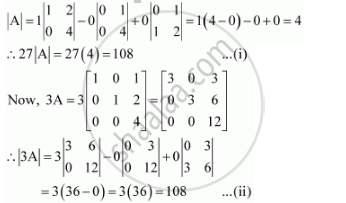Share

# If A=Matrix [(1,0,1),(0,1,2),(0,0,4)] Then Show that |3a| = 27|A| - CBSE (Commerce) Class 12 - Mathematics

ConceptDeterminants of Matrix of Order One and Two

#### Question

if A=[(1,0,1),(0,1,2),(0,0,4)] then show that |3A| = 27|A|

#### Solution

The given matrix is A = [(1,0,1),(0,1,2),(0,0,4)]

It can be observed that in the first column, two entries are zero. Thus, we expand along the first column (C1) for easier calculation.From equations (i) and (ii), we have:

|3A|  = 27|A|

Hence, the given result is proved.

Is there an error in this question or solution?

#### Video TutorialsVIEW ALL 

Solution If A=Matrix [(1,0,1),(0,1,2),(0,0,4)] Then Show that |3a| = 27|A| Concept: Determinants of Matrix of Order One and Two.
S